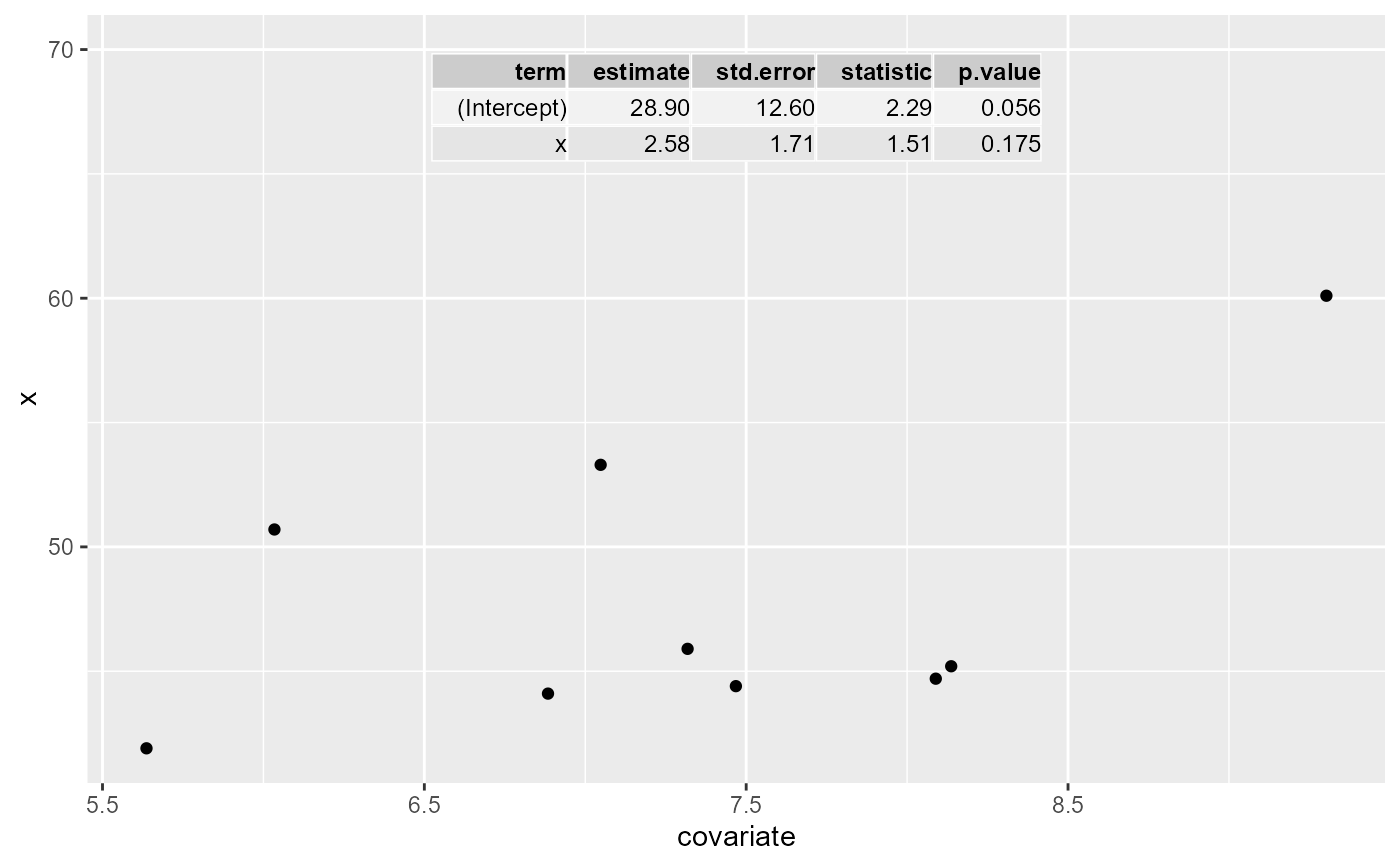stat_fit_tb fits a model and returns a "tidy" version of the model's summary or ANOVA table, using package 'broom'. The annotation is added to the plots in tabular form.

stat_fit_tb(mapping = NULL, data = NULL, geom = "table_npc",
method = "lm", method.args = list(formula = y ~ x),
tb.type = "fit.summary", tb.vars = NULL, digits = 3,
label.x = "center", label.y = "top", label.x.npc = NULL,
label.y.npc = NULL, position = "identity", na.rm = FALSE,
show.legend = FALSE, inherit.aes = TRUE, ...)

Arguments

mapping The aesthetic mapping, usually constructed with aes or aes_. Only needs to be set at the layer level if you are overriding the plot defaults. A layer specific dataset - only needed if you want to override the plot defaults. The geometric object to use display the data character. list of arguments to pass to method. character One of "fit.summary", "fit.anova" or "fit.coefs". character vector, optionally named, used to select and or rename the columns of the table returned. integer indicating the number of significant digits to be used. numeric Coordinates (in data units) to be used for absolute positioning of the output. If too short they will be recycled. numeric with range 0..1 or character. Coordinates to be used for positioning the output, expressed in "normalized parent coordinates" or character string. If too short they will be recycled. The position adjustment to use for overlapping points on this layer a logical indicating whether NA values should be stripped before the computation proceeds. logical. Should this layer be included in the legends? NA, the default, includes if any aesthetics are mapped. FALSE never includes, and TRUE always includes. If FALSE, overrides the default aesthetics, rather than combining with them. This is most useful for helper functions that define both data and aesthetics and shouldn't inherit behaviour from the default plot specification, e.g. borders. other arguments passed on to layer. This can include aesthetics whose values you want to set, not map. See layer for more details.

Computed variables

The output of tidy is returned as a single "cell" in a tibble (i.e. a tibble nested within a tibble). The returned data object contains a single, containing the result from a single model fit to all data in a panel. If grouping is present, it is ignored.

tidy for details on how the tidying of the resulst of model fits is done. See geom_table for details on how the formating and location of the table can be adjusted.

Examples

library(ggplot2)
# t-test example
x <- c(44.4, 45.9, 41.9, 53.3, 44.7, 44.1, 50.7, 45.2, 60.1)
group <- factor(c(rep("A", 4), rep("B", 5)))
my.df <- data.frame(x, group)

ggplot(my.df, aes(group, x)) +
geom_point() +
stat_fit_tb(method = "t.test",
tb.vars = c("italic(t)" = "estimate", "italic(P)" = "p.value"),
parse = TRUE)